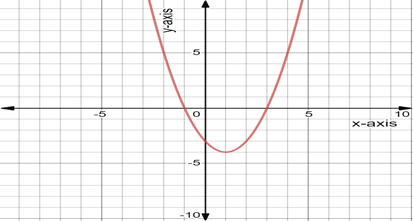# The blank in the statement, “the solution of the inequality x 2 − 2 x − 3 ≻ 0 are the x-coordinates of the points on the graph of y = x 2 − 2 x − 3 , that lie the x-axis ”.### Precalculus: Mathematics for Calcu...

6th Edition
Stewart + 5 others
Publisher: Cengage Learning
ISBN: 9780840068071### Precalculus: Mathematics for Calcu...

6th Edition
Stewart + 5 others
Publisher: Cengage Learning
ISBN: 9780840068071

#### Solutions

Chapter 1.9, Problem 2E
To determine

## To fill: The blank in the statement, “the solution of the inequality x2−2x−3≻0 are the x-coordinates of the points on the graph of y=x2−2x−3 , that lie          the x-axis ”.

Expert Solution

The complete statement of the inequality “ x22x30 are the x-coordinates of the points on the graph of y=x22x3 , that lie [1,3]by[0,0] the x-axis”.

### Explanation of Solution

Given information:

Consider the provided statement “ x22x30 are the intercepts of the graph y=x22x3 ”.

Consider the provide statement “ x22x3 are the intercepts of the graph y=x22x3 ”.

x -intercept is a point where a line crosses the x -axis.

y -intercepts is a point where a line crosses the y -axis.

So, the equation x22x3=0 . now check the equation for y=0 .

Here y=0 then the equation becomes:

x22x3=0x23xx3=0x(x3)+1(x3)=0(x+1)(x3)=0

Either x+1=0 or x2=0 :

Simplifies further as x=1 or x=3

The graph of the equation is open upward. Substitute the value of x in the equation x22x3=y .

Now for x=1 substitute the value of x in a given equation y=x22x3 .

y=x22x3y=12213y=0

Now for x=3 substitute the value of x in the given equation y=x22x3 .

y=x22x3y=32233y=963y=0

The graph of the given equation y=x22x3 is as:The parabola touches the x -axis at [1,3]by[0,0] , that means the parabola touches the x-axis at

1 and 3 .

Thus the complete statement, “the solution of the inequality x22x30 are the x-coordinates of the points on the graph of y=x22x3 , that lie [1,3]by[0,0] the x-axis ” .

### Have a homework question?

Subscribe to bartleby learn! Ask subject matter experts 30 homework questions each month. Plus, you’ll have access to millions of step-by-step textbook answers!